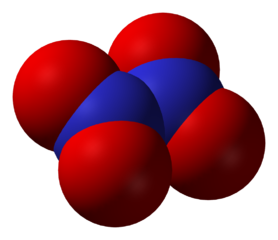10 minutes maximum! Can you do it in 5?

1. When a reaction is in dynamic equilibrium the rate of the forward reaction is the rate of the reverse reaction.

2. When a reaction is in dynamic equilibrium the concentrations of the reactants and products are always ...

• A.   equal
• B.   unequal with the reactant concentration greater than the product concentration
• C.   unequal with the product concentration greater than the reactant concentration
• D.   constant
3. The reaction of anhydrous copper(II)sulfate with water is an example of a reversible reaction:CuSO4 (s) + 5H2O (l) ⇌ CuSO4.5H2O (s) white blue

If the reaction moves in the forward direction you will observe ...

• A.   solid turning from white to blue
• B.   solid dissolving
• C.   solid turning from blue to white
• D.   condensation forming on the sides of the test tube

Q4-5
The production of ammonia in the Haber Process is an example of a reversible reaction:

 N2 (g) + 3H2 (g) ⇌ 2NH3 (g) ΔH = - 92.4kJ

Select the answer which states and gives a reason for the effect on the yield of ammonia (NH3) of …

4. ...increasing the temperature:

 Yield of Ammonia Reason A increases because the forward reaction is endothermic B increases because the reverse reaction is endothermic C decreases because the forward reaction is endothermic D decreases because the reverse reaction is endothermic

5. ...increasing the pressure:

 Yield of Ammonia Reason A increases because there are less moles of gas on the ‘products’ side B increases because there are less moles of gas on the ‘reactants’ side C decreases because there are less moles of gas on the ‘products’ side D decreases because there are less moles of gas on the ‘reactants’ side
6. The decomposition of dinitrogen tetroxide is an endothermic process as bonds are broken.

This is also a reversible reaction and the equation for this reaction is:

 N2O4 (g) ⇌ 2NO2 (g)

What would be the effect on the position of equilibrium of increasing the temperature and of increasing the pressure?Increasing the temperature Increasing the pressure A Moves to the left Moves to the left B Moves to the left Moves to the right C Moves to the right Moves to the left D Moves to the right Moves to the right
7. Hydrogen and chlorine react in a reversible reaction to produce hydrogen chloride:
 H2(g) + Cl2(g) ⇌ 2HCl(g)

If the pressure is increased on this equilibrium system, what will happen to the equilibrium position?

8. To achieve a high yield (of SO3), what conditions of temperature and pressure would be required?

 2SO2 + O2 ⇌ 2SO3 ΔH = -385 kJ

 Temperature Pressure A low low B low high C high low D high high

Q9-10:
Hydrogen is produced in industry by reacting methane with steam at 2 atm pressure and 1000 °C. The equation for the reaction is:

 CH4 (g) + H2O (g) ⇌ CO (g) + 3H2 (g) ΔH= +210 kJ

What is the effect on the concentrations of carbon monoxide and hydrogen in the equilibrium mixture of carrying out the reaction at...

9. ...500oC?

 Carbon Monoxide Concentration Hydrogen Concentration A increases decreases B increases increases C decreases decreases D decreases increases

10. ...10atm?

 Carbon Monoxide Concentration Hydrogen Concentration A increases decreases B increases increases C decreases decreases D decreases increases